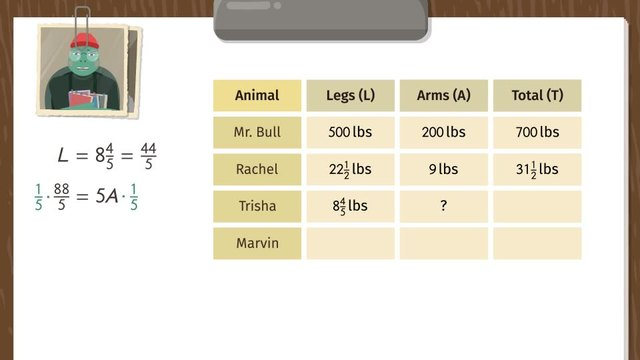# Equivalent Ratios Given Parts and WholesRate this video

Be the first to rate this video!

The authorSusan Sayfan

## Information about the videoEquivalent Ratios Given Parts and Wholes

After this lesson, you will be able to identify equivalent ratios and solve for every part of the proportion.

The lesson begins by teaching you to identify the constant of proportionality between parts and the whole ratio. It leads you to learn to use this constant to set-up an equation that will relate all parts. It concludes by solving for any part of a proportion through the use of that equation.

Learn about equivalent ratios by seeing Mr. Bull plan his trainees’ work-out in his gym.

This video introduces new concepts, notation, and vocabulary such as equivalent ratios (a set of paired quantities that is related by a proportional relationship) and constant of proportionality, usually expressed as k (which is the unit rate that will satisfy the equation that can represent the proportional relationship).

Before watching this video, you should already be familiar with ratio, proportion, proportional relationship, unit rates, ratio tables, operations on rational numbers, solving equations by cross multiplication, and solving equations by isolating variables.

After watching this video, you will be prepared to solve multi-step problems with ratios through percent, such as discounts, commissions, interest, taxes, fees, increases, decreases, and percent of error.

Common Core Standard(s) in focus: 6.RP.A.3.A A video intended for math students in the 6th grade